# GMAT Math : Calculating the endpoints of a line segment

## Example Questions

### Example Question #8 : Coordinate Geometry

The quadrilateral with vertices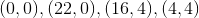is a trapezoid. What are the endpoints of its midsegment?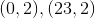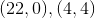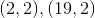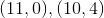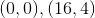Explanation:

The midsegment of a trapezoid is the segment whose endpoints are the midpoints of its legs - its nonparallel opposite sides. These two sides are the ones with endpoints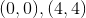and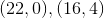. The midpoint of each can be found by taking the means of the- and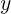-coordinates: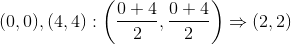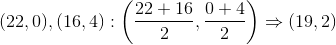The midsegment is the segment that has endpoints (2,2) and (19,2)

### Example Question #9 : Coordinate Geometry

The midpoint of a line segment with endpoints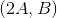and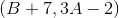is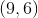. What is?It cannot be determined from the information given.Explanation:

If the midpoint of a line segment with endpointsandis, then by the midpoint formula,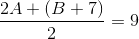and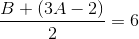.

The first equation can be simplified as follows: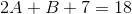or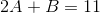The second can be simplified as follows: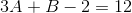or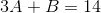This is a system of linear equations.can be calculated by subtracting: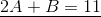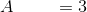### Example Question #10 : Coordinate Geometry

If the midpoint of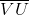is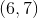and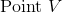is at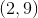, what are the coordinates of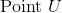?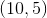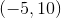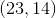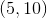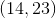Explanation:

Midpoint formula is as follows: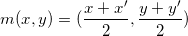In this case, we have x,y and the value of the midpoint. We need to findx' and y'

V is at (2,9) and the midpoint is at (6,7)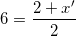and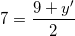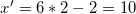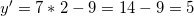So we have (10,5) as point U

### Example Question #1 : Calculating The Endpoints Of A Line Segment

A line segment has its midpoint at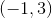and an endpoint at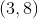.  What are the coordinates of the other endpoint?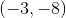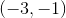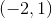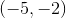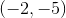Explanation:

Because we are given the midpoint and one of the endpoints, we know the x coordinate of the other endpoint will be the same distance away from the midpoint in the x direction, and the y coordinate of the other endpoint will be the same distance away from the midpoint in the y direction. Given two endpoints of the form: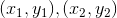The midpoint of these two endpoints has the coordinates: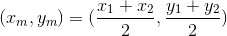Plugging in values for the given midpoint and one of the endpoints, which we can see is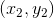because it lies to the right of the midpoint, we can solve for the other endpoint as follows: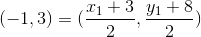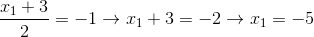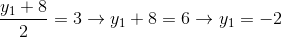So the other endpoint has the coordinates### Example Question #2 : Calculating The Endpoints Of A Line Segment

Consider segment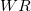with endpoint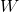at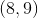. If the midpoint ofcan be found at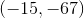, what are the coordinates of point?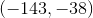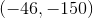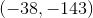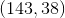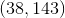Explanation:

Recall midpoint formula: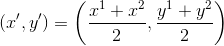In this case we have (x'y') and one of our other (x,y) points.

Plug and chug: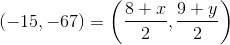If you make this into two equations and solve you get the following.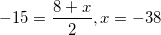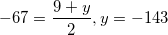Tired of practice problems?

Try live online GMAT prep today.